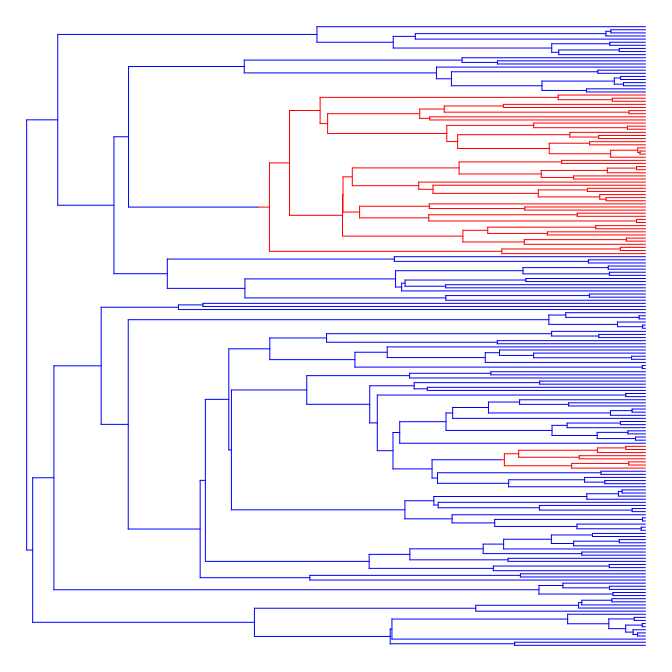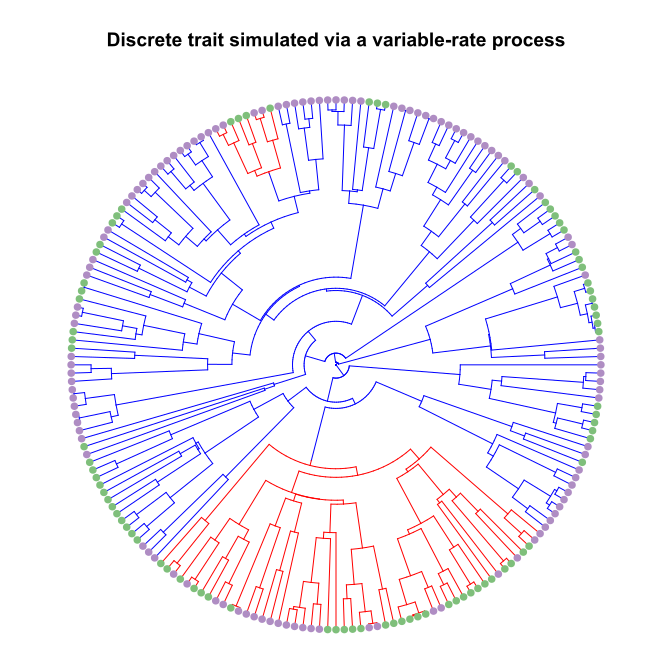## Saturday, February 3, 2018

### Function to simulate variable-rate Mk model mapped onto the tree

A little while ago I posted a function to fit multiple Mk models to different painted 'regimes' on the tree.

This method differs from others such as the 'covarion' model of Penny et al. (2001) or the related hidden rates model of Beaulieu et al. (2013) in that it treats the regimes as 'known' - rather than integrating over uncertainty in their painting. Thus, the approach is more appropriate for comparing among a limited number of a priori hypotheses - such as a difference in rate between clades or across time periods (such as geological eras), rather than for testing for a relationship between two or more discrete traits or for trying to identify changes in the rate of character evolution for a single discrete trait, absent an a priori hypothesis regarding how the rate may have changed.

I'm trying to write a short note describing this method, but first I need a simulator for the model. In a prior post, I simulated under a variable-rate model merely by stretching the branch lengths of the tree. Unfortunately, this only works if Q2 is a scalar multiple of Q1. For more complex scenarios in which the various Qi we'd like to simulate are not proportional we'll need a tool that is a tiny bit more sophisticated.

Using the phytools `"simmap"` object class to encode our paintings, the way I decided to do this was first by converting our `"simmap"` object to an ordinary `"phylo"` object with singleton nodes instead of mappings along edges. This way, we can only have one regime per edge. Then I proceeded across all the edges of the tree with singletons and for each edge computed P=expm(Qi×t) in which Qi indicates the correct transition matrix for that edge, t is the length of the edge, expm is the matrix exponential, and P is the matrix of transition probabilities. Next, I proceeded to carry out a single preorder tree traversal in which every node is visited, a character state is assigned using the state at the parent node and the computed matrix of transition probabilities, P, and then the algorithm proceeds to the daughters of the current node until all nodes have been visited.

Here is what that code looks like:

``````sim.multiMk<-function(tree,Q,anc=NULL,...){
ss<-rownames(Q[])
tt<-map.to.singleton(reorder(tree))
P<-vector(mode="list",length=nrow(tt\$edge))
for(i in 1:nrow(tt\$edge))
P[[i]]<-expm(Q[[names(tt\$edge.length)[i]]]*tt\$edge.length[i])
if(is.null(anc)) anc<-sample(ss,1)
STATES<-matrix(NA,nrow(tt\$edge),2)
root<-Ntip(tt)+1
STATES[which(tt\$edge[,1]==root),1]<-anc
for(i in 1:nrow(tt\$edge)){
new<-ss[which(rmultinom(1,1,P[[i]][STATES[i,1],])[,1]==1)]
STATES[i,2]<-new
ii<-which(tt\$edge[,1]==tt\$edge[i,2])
if(length(ii)>0) STATES[ii,1]<-new
}
x<-as.factor(
setNames(sapply(1:Ntip(tt),function(n,S,E) S[which(E==n)],
S=STATES[,2],E=tt\$edge[,2]),tt\$tip.label))
x
}
``````

For completeness, here's an equivalent simulator for a single Q matrix:

``````sim.Mk<-function(tree,Q,anc=NULL,...){
ss<-rownames(Q)
tt<-reorder(tree)
P<-vector(mode="list",length=nrow(tt\$edge))
for(i in 1:nrow(tt\$edge))
P[[i]]<-expm(Q*tt\$edge.length[i])
if(is.null(anc)) anc<-sample(ss,1)
STATES<-matrix(NA,nrow(tt\$edge),2)
root<-Ntip(tt)+1
STATES[which(tt\$edge[,1]==root),1]<-anc
for(i in 1:nrow(tt\$edge)){
new<-ss[which(rmultinom(1,1,P[[i]][STATES[i,1],])[,1]==1)]
STATES[i,2]<-new
ii<-which(tt\$edge[,1]==tt\$edge[i,2])
if(length(ii)>0) STATES[ii,1]<-new
}
x<-as.factor(
setNames(sapply(1:Ntip(tt),function(n,S,E) S[which(E==n)],
S=STATES[,2],E=tt\$edge[,2]),tt\$tip.label))
x
}
``````

For fun, let's imagine the following regimes:

``````library(phytools)
colors<-setNames(c("blue","red"),0:1)
plot(tree,lwd=1,colors=colors,ftype="off")
``````& then let's simulate two characters, x & y on this tree in which x evolved via a variable-rate process, whereas y evolved under a single set of transition rates:

``````Qx<-setNames(
list(matrix(c(-0.5,0.5,0.5,-0.5),2,2,dimnames=list(letters[1:2],
letters[1:2])),
matrix(c(-5,5,5,-5),2,2,dimnames=list(letters[1:2],
letters[1:2]))),0:1)
Qx
``````
``````## \$`0`
##      a    b
## a -0.5  0.5
## b  0.5 -0.5
##
## \$`1`
##    a  b
## a -5  5
## b  5 -5
``````
``````Qy<-matrix(c(-1,1,1,-1),2,2,dimnames=list(letters[1:2],letters[1:2]))
Qy
``````
``````##    a  b
## a -1  1
## b  1 -1
``````
``````x<-sim.multiMk(tree,Qx)
plot(tree,colors,lwd=1,ftype="off",type="fan",mar=c(1.1,1.1,4.1,1.1))
par(fg="transparent")
tiplabels(pie=to.matrix(x,letters[1:2]),
piecol=RColorBrewer::brewer.pal(n=3,"PRGn")[c(1,3)],
cex=0.3)
par(fg="black")
title(main="Discrete trait simulated via a variable-rate process")
````````````y<-sim.Mk(tree,Qy)
plot(tree,colors,lwd=1,ftype="off",type="fan",mar=c(1.1,1.1,4.1,1.1))
par(fg="transparent")
tiplabels(pie=to.matrix(y,letters[1:2]),
piecol=RColorBrewer::brewer.pal(n=3,"PRGn")[c(1,3)],
cex=0.3)
par(fg="black")
title(main="Discrete trait simulated via a constant-rate process")
``````